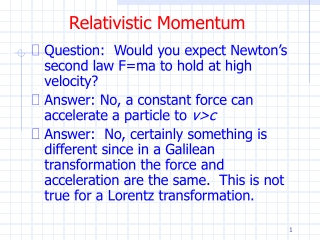DownloadDownload PresentationRelativistic Momentum

Relativistic Momentum

Download PresentationRelativistic Momentum

- - - - - - - - - - - - - - - - - - - - - - - - - - - E N D - - - - - - - - - - - - - - - - - - - - - - - - - - -
Presentation Transcript

1. Relativistic Momentum • Question: Would you expect Newton’s second law F=ma to hold at high velocity? • Answer: No, a constant force can accelerate a particle to v>c • Answer: No, certainly something is different since in a Galilean transformation the force and acceleration are the same. This is not true for a Lorentz transformation.

2. Relativistic Momentum • Galilean transformation • We showed a=a’and F=F’ • Lorentz transformation • We might try starting with F=dp/dt

3. Newtonian Relativity • Note Newton’s laws are valid in both frames • The force and acceleration are the same in both frames • There is no way to detect which frame is moving and which is at rest

4. Relativistic Momentum • Frank (F) is in K with a ball of mass m • Mary (M) is in K’ with a ball of mass m • Frank throws his ball along y with velocity u0 • Mary throws her ball along –y’ with velocity u0 • The balls collide elastically

5. Relativistic Momentum

6. Relativistic Momentum • Frank sees the momentum change of his ball • Frank sees for Mary’s ball • Frank sees the momentum change of Mary’s ball

7. Addition of Velocities • Recall the addition of velocities in special relativity

8. Relativistic Momentum • Momentum is not conserved in the y direction! • Because we strongly believe in the conservation of momentum, let’s modify the definition of momentum

9. Relativistic Momentum • Frank sees the momentum change of his ball • Frank sees the momentum change of Mary’s ball

10. Relativistic Momentum • Thus Frank sees that momentum is conserved in the x and y directions using • Likewise Mary would see that momentum conservation holds in her frame as well

11. Relativistic Momentum • Notes • Unfortunately γ is used for both • Usually we write them out when they both come into play • In these equations m=m0 is the rest mass • Sometime we interpret γm as the relativistic mass but that is not standard

12. Relativistic Energy • Let’s assume that the relativistically correct form of Newton’s law is given by • The validity of this assumption can be determined by examining its consequences • Aside, are Newton’s first and third laws relativistically correct?

13. Relativistic Energy • In classical mechanics the work done by a force in moving a particle from one position to another equals the change in kinetic energy

14. Relativistic Energy • We can evaluate the integral by writing

15. Relativistic Energy • Then • Thus T = γmc2 – mc2

16. Relativistic Energy • Now at low speeds we can use the binomial expansion • To find as expected

17. Relativistic Energy • We define the total energy E • E = kinetic energy plus rest energy • E = T + mc2 • E = γmc2

18. Relativistic Energy • We also have for E • Thus • E2=p2c2 + m2c4 • E = mc2 for p=0